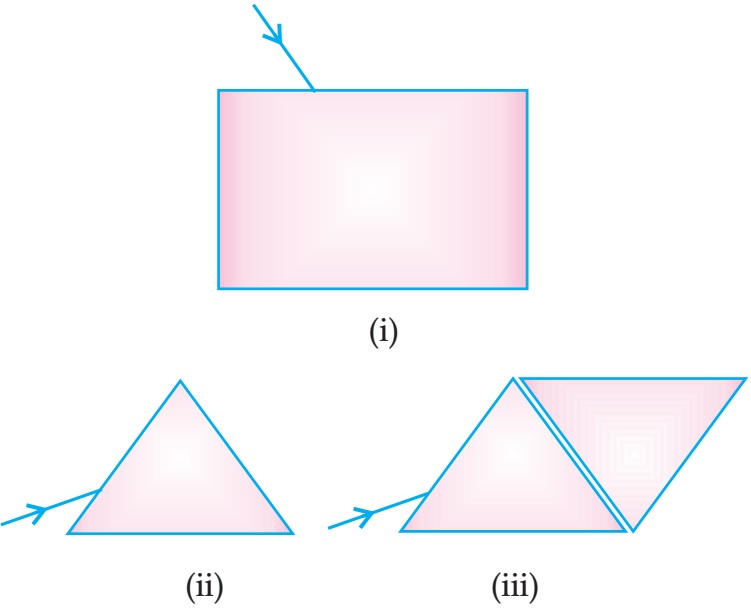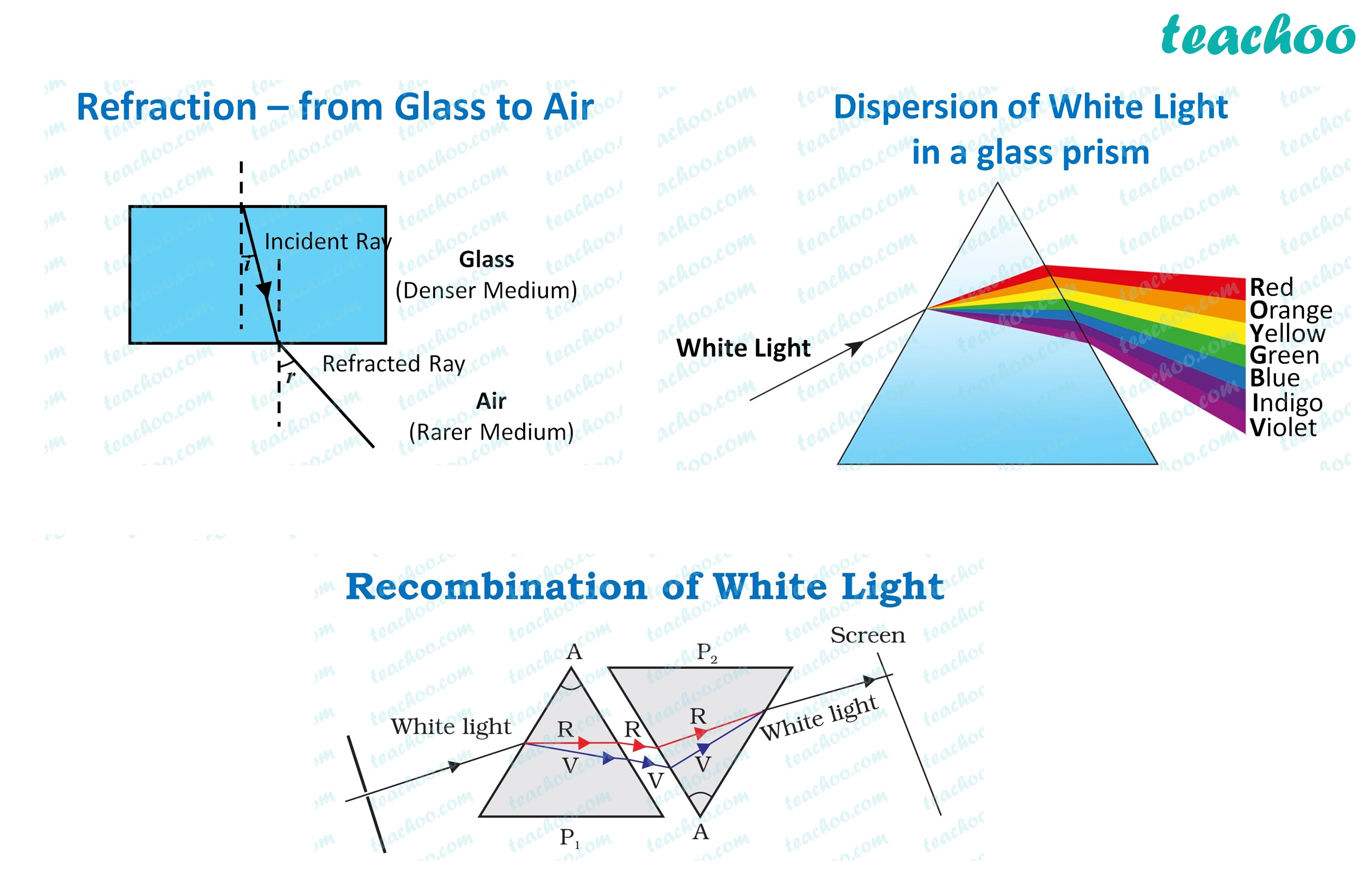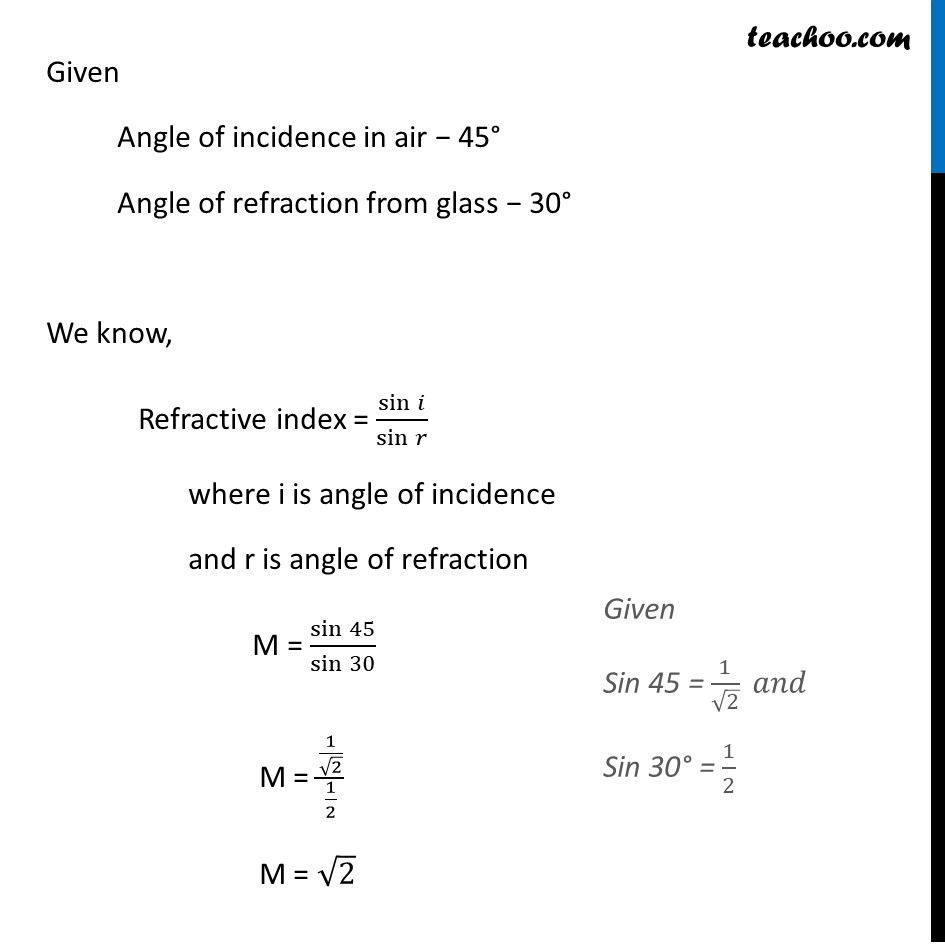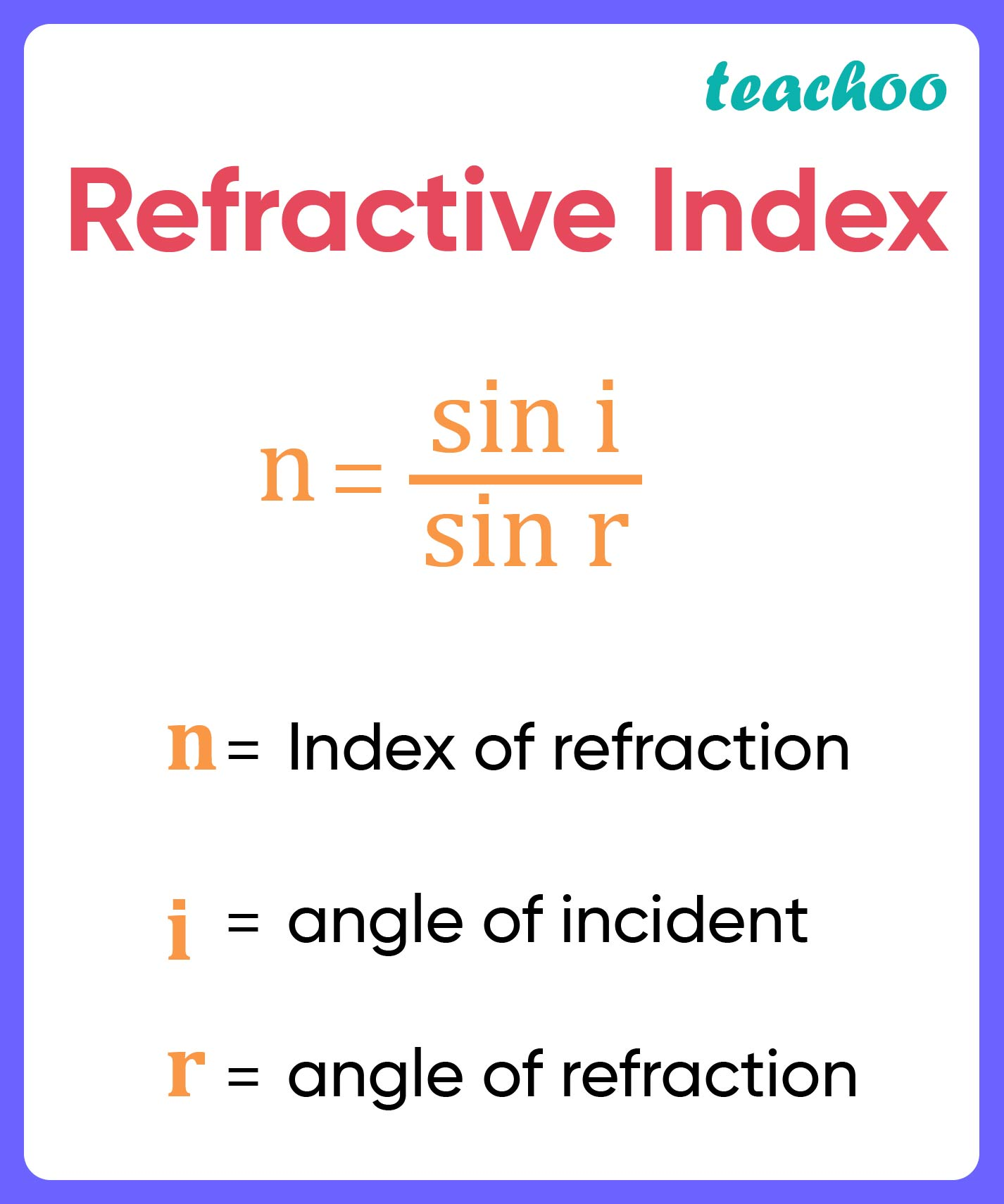Case Based Questions (MCQ)

Class 10
Chapter 10 Class 10 - Light - Reflection and Refraction

##googletag.cmd.push(function() { googletag.display('div-gpt-ad-1669298377854-0'); });

### (iv) No similar emergent beamsIn all 3 cases

• In (i) the emergent beam is white and laterally displaced .
• In (ii) emergent beam is a spectrum of seven colours bent in different angles.
• In (iii) the emergent beam from the second prism is  white only .

Similarity between (i) and (iii) as both emergent  rays are white in colour

So, the correct answer is (ii) (i) and (iii)

### (iv) 1

Ans.So, the correct answer is (i) √2

### (iv) The light chooses the path with minimum time, as it changes its medium.

Ans.

• Speed of light is different in different media - Speed of light is less in glass as compared to in air. Hence, we can say that the speed of light is different in different media.
Option (i) is correct

• Light changes its path because light only  travels in a straight line - Light doesn't change it’s path , it just bends to an angle while traveling in different media.
Option (ii) is incorrect.

• Speed of light is dependent on the medium  through which it is passing - This is true .
Option (iii) is correct

• The light chooses the path with minimum time, as it changes its medium - By definition, light always chooses the path which will take the least time.
Option (iv) is also correct

So, the correct answer is (ii)

### (iv) μ m

Ans.

Refractive index is the ratio of sine of angle of incidence to the sine of angle of refraction which is a constant , hence no unit .So, the correct answer is (iii) No unit

### (iv) Diamond

Ans.

• Light travel fastest where there no obstructions
• In vacuum, there is no obstructions
• Hence, light travel fastest in vacuum

So, the correct answer is (ii) vacuum.

Learn in your speed, with individual attention - Teachoo Maths 1-on-1 Class